# Visualization walkthrough using ggplot2 Library in R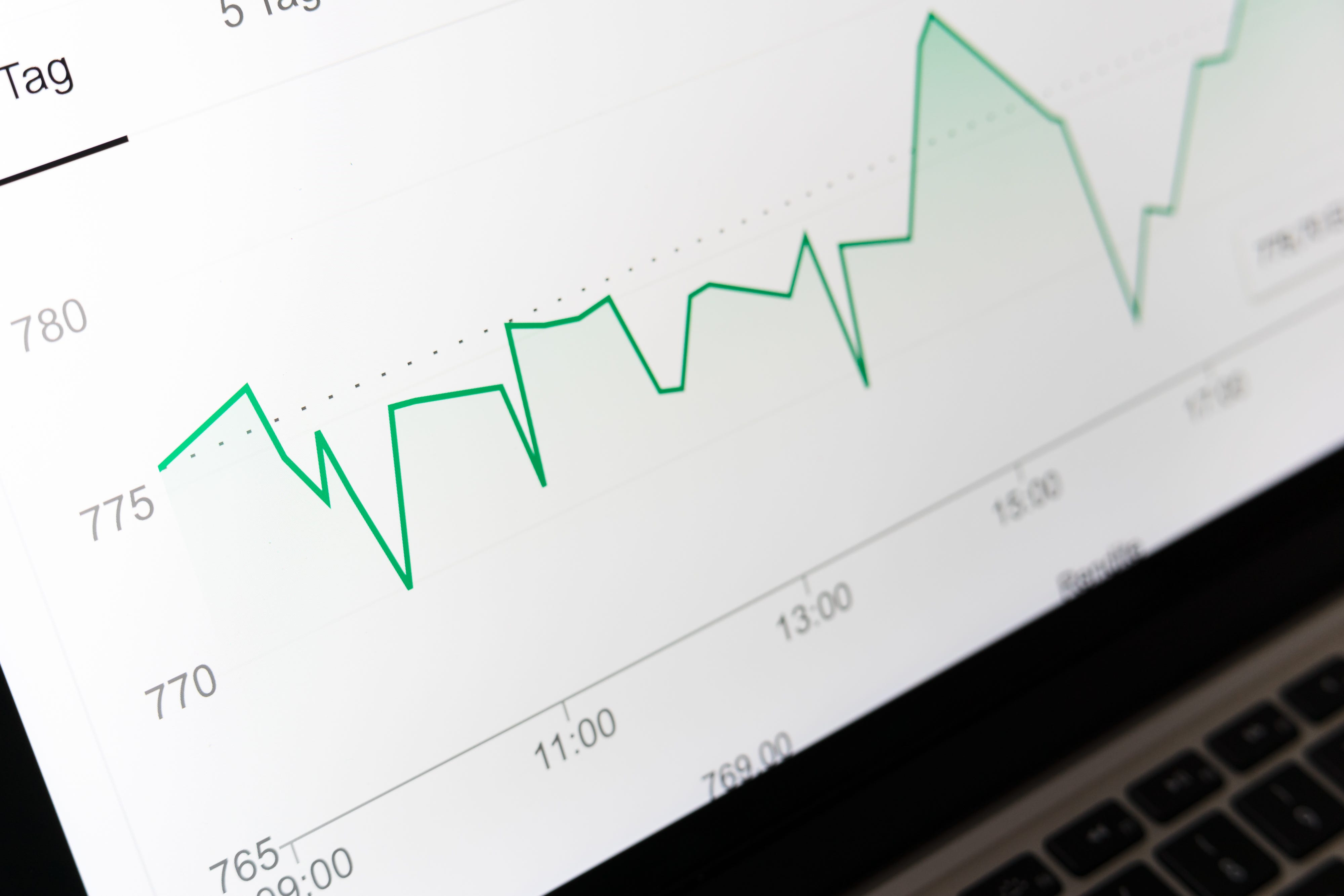Photo by Markus Winkler on Unsplash

This post is for beginners who are looking to use the ggplot2 library in R for Data Visualization

For our example, we will get our HDB dataset from www.data.gov.sg.

We will save our dataset in our local as “HDB_DATASET.csv” in CSV format.

Pre-requisites: R -Studio and R should be installed on your PC.

Launch R Studio and load the hdb data set

hdb_data <- read.csv(file = “HDB_DATASET.csv”, stringsAsFactors=TRUE)
head(hdb_data)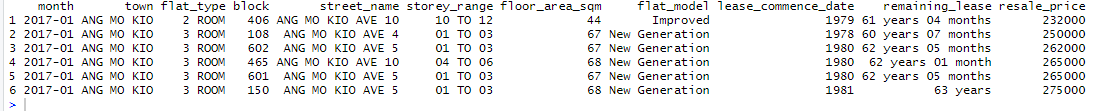head(hdb_data)

Check if there are any missing values or null values

cleaned_data<-hdb_data
which(is.na(cleaned_data),arr.ind=TRUE)

if there are any missing values, you can clean the data using techniques like Deletion — Listwise, Pairwise, Imputation -Mean, Median, Mode.

Once our data cleaning is completed, we have the dataset ready for visualization in R.

cleaned_data

Now, we will try to visualize the data to see most HDB sold across towns in Singapore between 2017 to 2020.

We will aggregate the resale price by town

average_resale_price<- aggregate(cleaned_data\$resale_price,FUN = mean,by=list(cleaned_data\$town))

we will also count the no of the resale transaction by town

count_table<- cleaned_data %>% group_by(cleaned_data\$town) %>% summarise(count=n())

We will do a quick sorting by descending order using count

average_resale_price <- average_resale_price %>% cbind(count_table\$count) %>% arrange(Group.1,desc(ave(count_table\$count)))
head(average_resale_price)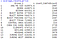average_resale_price

We will rename the columns for clarity

names(average_resale_price)<-”Town”
names(average_resale_price)<-”AveragePrice”
names(average_resale_price)<-”Count”

We can create the bar plot using town and count column like below using ggplot2 library

library(ggplot2)

dashplot1<-ggplot(average_resale_price,aes(x=reorder(Town,-Count),y=Count))+labs(x=”Singapore Towns”,y=”No of HDB(s) sold”)+geom_bar(stat=”identity”)

As you can see the labels in x-axis are overlapping. We can fix this like below

dashplot1<-dashplot1+theme(axis.text.x = element_text(angle=90),)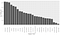dashplot1

So far we are good, but for keeping it simple we can remove the gridlines and add axis border lines like below

dashplot1<-dashplot1+theme(panel.grid.major = element_blank(),panel.grid.minor = element_blank(),panel.background = element_blank(),axis.line = element_line(colour = “black”))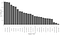dashplot1

To have a little appealing look we can add a simple color palette which is fading out in nature like below

cp<-colorRampPalette(c(“brown”,”grey”))length(average_resale_price\$Town))

dashplot1+geom_col(fill=cp)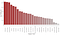dashplot1

Finally, we can add a title for the chart like below.

dashplot1 <-dashplot1+labs(title=”Most HDB sold across towns in Singapore between 2017 to 2020")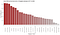dashplot1

Similarly, you can create other visual charts (stacked bar) in R using ggplot2 library.

most_sold_hdb_type <-c(“4 ROOM”,”5 ROOM”)
total_asp<- cleaned_data %>% filter(flat_type %in% most_sold_hdb_type)
total_asp<- aggregate(total_asp\$resale_price,FUN = mean,by=list(total_asp\$town))

names(total_asp)<-”Town”
names(total_asp)<-”Average”

total_asp <-arrange(total_asp, desc(Average))

cp<-colorRampPalette(c(“red”,”green”))(length(total_asp\$Town));
dashplot2<-ggplot(total_asp,aes(reorder(Town,-Average),Average)) +
labs(x=”Singapore Towns”,y=”Avg. price”) +
geom_col(fill=cp) + theme(axis.text.x = element_text(angle=90),) +
scale_y_continuous(labels = dollar) + theme(panel.grid.major = element_blank(),panel.grid.minor = element_blank(),panel.background = element_blank(),axis.line = element_line(colour = “black”))

plot_insight2 <- dashplot2 + labs(title=”4-Room/5-Room Avg. Resale Price across towns in Singapore”)
plot_insight2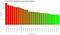plot_insight2

hdb_north_west<- cleaned_data %>% filter(town %in% c(“SENGKANG”,”WOODLANDS”,”JURONG WEST”))

hdb_north_west <- hdb_north_west %>% group_by(hdb_north_west\$town,hdb_north_west\$flat_type) %>% summarise(count=n())

names(hdb_north_west) <- “Town”
names(hdb_north_west) <- “FlatType”
names(hdb_north_west) <- “Count”
hdb_north_west

plot_insight3<-ggplot(hdb_north_west,aes(x=reorder(Town,Count),y=Count,fill=reorder(FlatType,-Count))) +
geom_bar(stat=”identity”,color=”black”)+
labs(y=”No of HDB(s) sold”) +
scale_fill_discrete(name=”Flat type”) +
theme(axis.text.x = element_text(angle=90),axis.title.x = element_blank())+theme(panel.grid.major = element_blank(),panel.grid.minor = element_blank(),panel.background = element_blank(),axis.line = element_line(colour = “black”))

plot_insight3 <- plot_insight3 + labs(title=” Flat Types sold in top 3 towns in Singapore “)
plot_insight3

Documentation References: https://www.rdocumentation.org/packages/ggplot2/versions/3.3.2

Hope you like this example. Thanks.

Studying Data. Django and Python Enthusiast.

## More from Syed Jameer

Studying Data. Django and Python Enthusiast.